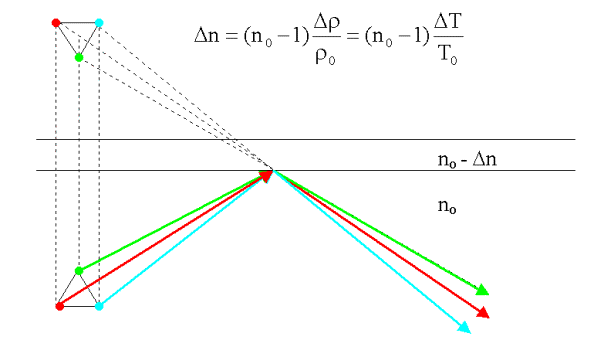# What is the Fermat Principle

## Snellius’s law of refraction

Fermat’s principle

The propagation of the light takes place between two points A and B of a medium on that path for which the required time is a minimum: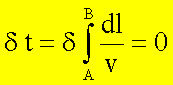Because n = c / v one obtains with ds = n.dl as optical path length:With the help of Fermat’s principle, for example, Snellius’s law of refraction can be derived: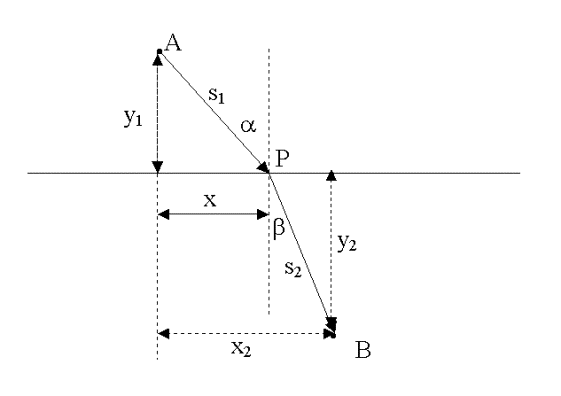The light beam should go from point A to point B. The position of point P is varied until the time to run through the distance

A-P-B becomes a minimum.

The total time results in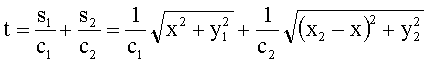The time should be minimal, i.e.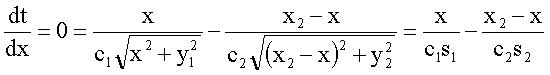With sina = x / s1 and sinb = (x2-x) / s2 that follows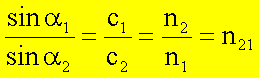If the refractive index changes continuously, e.g. in warmed layers of air, a curvature of light takes place.

In the case of a very shallow incidence on an optically thinner medium (heated air), a quasi total reflection.